# sklearn.linear_model.LinearRegression¶

class sklearn.linear_model.LinearRegression(fit_intercept=True, normalize=False, copy_X=True, n_jobs=None)[source]

Ordinary least squares Linear Regression.

Parameters: fit_intercept : boolean, optional, default True whether to calculate the intercept for this model. If set to False, no intercept will be used in calculations (e.g. data is expected to be already centered). normalize : boolean, optional, default False This parameter is ignored when fit_intercept is set to False. If True, the regressors X will be normalized before regression by subtracting the mean and dividing by the l2-norm. If you wish to standardize, please use sklearn.preprocessing.StandardScaler before calling fit on an estimator with normalize=False. copy_X : boolean, optional, default True If True, X will be copied; else, it may be overwritten. n_jobs : int or None, optional (default=None) The number of jobs to use for the computation. This will only provide speedup for n_targets > 1 and sufficient large problems. None means 1 unless in a joblib.parallel_backend context. -1 means using all processors. See Glossary for more details. coef_ : array, shape (n_features, ) or (n_targets, n_features) Estimated coefficients for the linear regression problem. If multiple targets are passed during the fit (y 2D), this is a 2D array of shape (n_targets, n_features), while if only one target is passed, this is a 1D array of length n_features. intercept_ : array Independent term in the linear model.

Notes

From the implementation point of view, this is just plain Ordinary Least Squares (scipy.linalg.lstsq) wrapped as a predictor object.

Examples

>>> import numpy as np
>>> from sklearn.linear_model import LinearRegression
>>> X = np.array([[1, 1], [1, 2], [2, 2], [2, 3]])
>>> # y = 1 * x_0 + 2 * x_1 + 3
>>> y = np.dot(X, np.array([1, 2])) + 3
>>> reg = LinearRegression().fit(X, y)
>>> reg.score(X, y)
1.0
>>> reg.coef_
array([1., 2.])
>>> reg.intercept_
3.0000...
>>> reg.predict(np.array([[3, 5]]))
array([16.])


Methods

 fit(X, y[, sample_weight]) Fit linear model. get_params([deep]) Get parameters for this estimator. predict(X) Predict using the linear model score(X, y[, sample_weight]) Returns the coefficient of determination R^2 of the prediction. set_params(**params) Set the parameters of this estimator.
__init__(fit_intercept=True, normalize=False, copy_X=True, n_jobs=None)[source]
fit(X, y, sample_weight=None)[source]

Fit linear model.

Parameters: X : array-like or sparse matrix, shape (n_samples, n_features) Training data y : array_like, shape (n_samples, n_targets) Target values. Will be cast to X’s dtype if necessary sample_weight : numpy array of shape [n_samples] Individual weights for each sample New in version 0.17: parameter sample_weight support to LinearRegression. self : returns an instance of self.
get_params(deep=True)[source]

Get parameters for this estimator.

Parameters: deep : boolean, optional If True, will return the parameters for this estimator and contained subobjects that are estimators. params : mapping of string to any Parameter names mapped to their values.
predict(X)[source]

Predict using the linear model

Parameters: X : array_like or sparse matrix, shape (n_samples, n_features) Samples. C : array, shape (n_samples,) Returns predicted values.
score(X, y, sample_weight=None)[source]

Returns the coefficient of determination R^2 of the prediction.

The coefficient R^2 is defined as (1 - u/v), where u is the residual sum of squares ((y_true - y_pred) ** 2).sum() and v is the total sum of squares ((y_true - y_true.mean()) ** 2).sum(). The best possible score is 1.0 and it can be negative (because the model can be arbitrarily worse). A constant model that always predicts the expected value of y, disregarding the input features, would get a R^2 score of 0.0.

Parameters: X : array-like, shape = (n_samples, n_features) Test samples. For some estimators this may be a precomputed kernel matrix instead, shape = (n_samples, n_samples_fitted], where n_samples_fitted is the number of samples used in the fitting for the estimator. y : array-like, shape = (n_samples) or (n_samples, n_outputs) True values for X. sample_weight : array-like, shape = [n_samples], optional Sample weights. score : float R^2 of self.predict(X) wrt. y.
set_params(**params)[source]

Set the parameters of this estimator.

The method works on simple estimators as well as on nested objects (such as pipelines). The latter have parameters of the form <component>__<parameter> so that it’s possible to update each component of a nested object.

Returns: self

## Examples using sklearn.linear_model.LinearRegression¶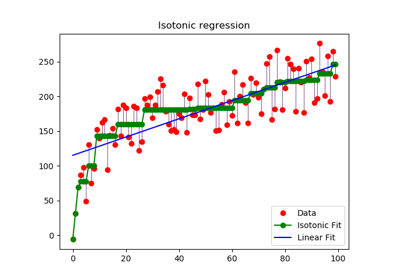Isotonic Regression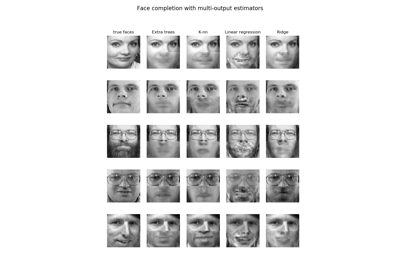Face completion with a multi-output estimators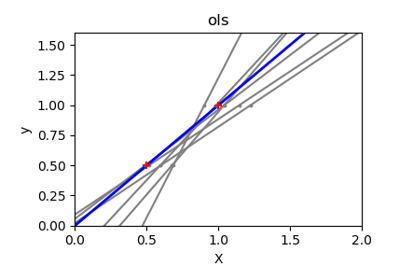Ordinary Least Squares and Ridge Regression Variance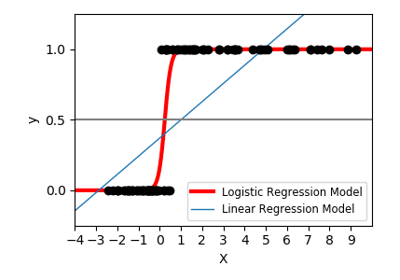Logistic function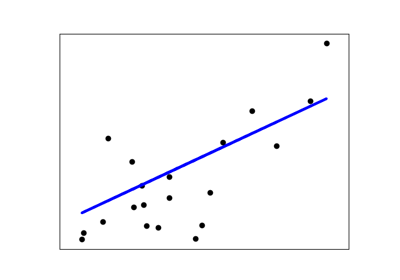Linear Regression Example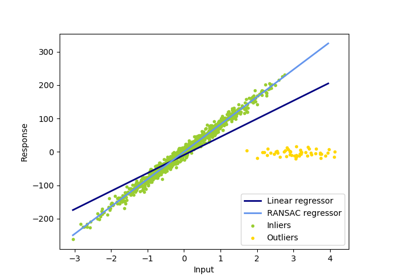Robust linear model estimation using RANSAC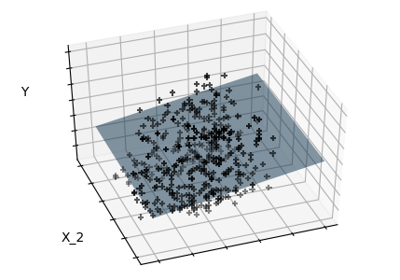Sparsity Example: Fitting only features 1 and 2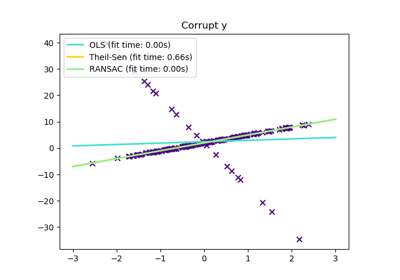Theil-Sen Regression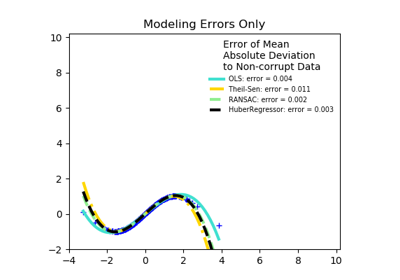Robust linear estimator fitting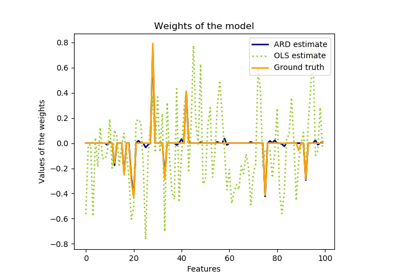Automatic Relevance Determination Regression (ARD)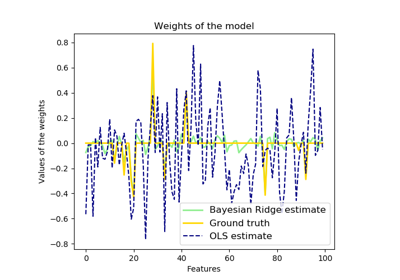Bayesian Ridge Regression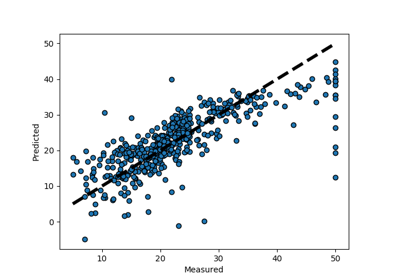Plotting Cross-Validated Predictions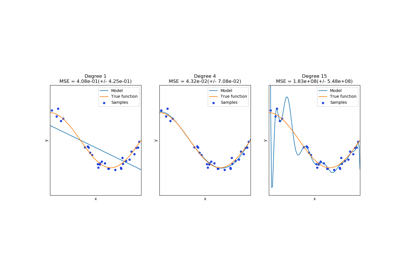Underfitting vs. Overfitting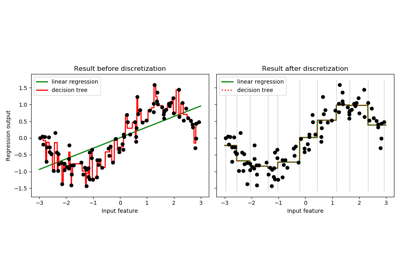Using KBinsDiscretizer to discretize continuous features